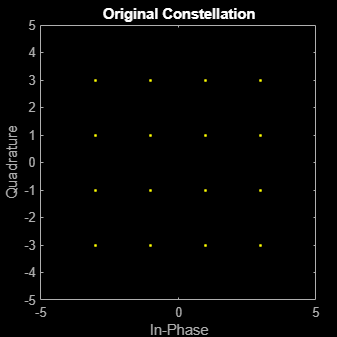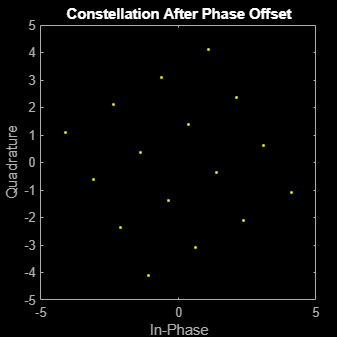# comm.PhaseFrequencyOffset

Apply phase and frequency offsets to input signal

## Description

The `PhaseFrequencyOffset` object applies phase and frequency offsets to an incoming signal.

To apply phase and frequency offsets to the input signal:

1. Define and set up your phase frequency offset object. See Construction.

2. Call `step` to apply phase and frequency offsets to the input signal according to the properties of `comm.PhaseFrequencyOffset`. The behavior of `step` is specific to each object in the toolbox.

Note

Starting in R2016b, instead of using the `step` method to perform the operation defined by the System object™, you can call the object with arguments, as if it were a function. For example, ```y = step(obj,x)``` and `y = obj(x)` perform equivalent operations.

## Construction

`H = comm.PhaseFrequencyOffset` creates a phase and frequency offset System object, `H`. This object applies phase and frequency offsets to an input signal.

`H = comm.PhaseFrequencyOffset(Name,Value)` creates a phase and frequency offset object, `H`, with each specified property set to the specified value. You can specify additional name-value pair arguments in any order as (`Name1`,`Value1`,...,`NameN`,`ValueN`).

## Properties

 `PhaseOffset` Phase offset Specify the phase offset in degrees. The default is `0`. If the `step` method input is an M-by-N matrix, the `PhaseOffset` property can be set to a numeric scalar, an M-by-1, or 1-by-N numeric vector, or an M-by-N numeric matrix. For more information, see Interdependent Property-Input Dimensions. Tunable: Yes `FrequencyOffsetSource` Source of frequency offset Specify the source of the frequency offset as one of `Property` | `Input port`. The default is `Property`. If you set this property to `Property`, you can specify the frequency offset using the `FrequencyOffset` property. If you set this property to `Input port`, you specify the frequency offset as a step method input. `FrequencyOffset` Frequency offset Specify the frequency offset in Hertz. The default is `0`. If the `step` method input is an M-by-N matrix, then the `FrequencyOffset` property is a numeric scalar, an M-by-1, or 1-by-N numeric vector, or an M-by-N numeric matrix. For more information, see Interdependent Property-Input Dimensions. This property applies when you set the `FrequencyOffsetSource` property to `Property`. Tunable: Yes `SampleRate` Sample rate Specify the sample rate of the input samples in seconds as a double-precision, real, positive scalar value. The default is `1`.

## Methods

 step Apply phase and frequency offsets to input signal
Common to All System Objects
`release`

Allow System object property value changes

## Examples

collapse all

Introduce a phase offset to a 16-QAM signal and view its effect on the constellation.

Create a phase frequency offset System object™. Set the phase offset to 30 degrees.

`pfo = comm.PhaseFrequencyOffset('PhaseOffset',30);`

Generate random symbols and apply 16-QAM modulation.

```M = 16; data = (0:M-1)'; modData = qammod(data,M); ```

Plot the 16-QAM constellation.

```scatterplot(modData); title(' Original Constellation') xlim([-5 5]) ylim([-5 5])```Introduce a phase offset using `pfo` and plot the offset constellation. Note that it has been shifted 30 degrees.

```impairedData = pfo(modData); scatterplot(impairedData); title('Constellation after phase offset') xlim([-5 5]) ylim([-5 5])```expand all

## Algorithms

If the input signal is u(t), then the output signal is

`$y\left(t\right)=u\left(t\right)\cdot \left(\mathrm{cos}\left(2\pi {\int }_{0}^{t}f\left(\tau \right)d\tau +\phi \left(t\right)\right)+j\mathrm{sin}\left(2\pi {\int }_{0}^{t}f\left(\tau \right)d\tau +\phi \left(t\right)\right)\right)\text{,}$`

where f(t) is the frequency offset, and φ(t) is the phase offset.

The discrete-time output is given by

where i > 0, and Δt is the sample time.